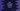# C++ program to print A to Z in different ways## C++ program to print A to Z:

In this C++ program, we will learn how to print the alphabets i.e. from A to Z. We can use a loop to print these characters.

We will learn different ways to solve this problem and different ways to print the characters.

## Example 1: C++ program to print from A to Z using a for loop:

Let’s try this by using a for loop. A for loop is defined as like below:

``````for(initial_condition; loop_condition; end_condition){

}``````

The initial_condition is the initial condition, i.e. we can define a variable here. loop_condition is the condition to run the loop, the loop will keep running till this return true. end_condition is a condition to run after each execution.

The below program uses a for loop to print the characters from A to Z:

``````#include <iostream>
using namespace std;

int main()
{
for (char c = 'A'; c <= 'Z'; c++)
{
cout << c << ' ';
}
}``````

It will run from c = ‘A’ to c = ‘Z’ and on each iteration, it moves to the next character.

It will print the characters in one line. If you run this program, it will print the below output:

``A B C D E F G H I J K L M N O P Q R S T U V W X Y Z ``

## Example 2: C++ program to print each character in new line:

We can also change this program to print each character in a new line:

``````#include <iostream>
using namespace std;

int main()
{
for (char c = 'A'; c <= 'Z'; c++)
{
cout << c << endl;
}
}``````

You need to replace the empty character with endl to add one new line after each character it prints.

It will print:

``````A
B
C
...``````

## Example 3: C++ program to print from A to Z separated by a comma:

We can also modify this program to print A to Z with a comma as the separator. The only thing we need to keep in mind that we don’t want a comma at the end.

``````#include <iostream>
using namespace std;

int main()
{
for (char c = 'A'; c <= 'Z'; c++)
{
cout << c;

c == 'Z' ? cout << endl : cout << ',';
}
}``````
• It is printing the character first.
• If the printed character is Z, it adds one new line, else it adds one ,.

If you run this program, it will give one output as like below:

``A,B,C,D,E,F,G,H,I,J,K,L,M,N,O,P,Q,R,S,T,U,V,W,X,Y,Z``

You can change comma with any other character as well.

## Example 4: C++ program to print from A to Z using numbers:

We can also use the ASCII values of these characters. For example, the ASCII of A is 65 and ASCII of Z is 90. We can change the program as like below:

``````#include <iostream>
using namespace std;

int main()
{
for (char c = 65; c <= 90; c++)
{
cout << c << ' ';
}
}``````

Note that we are still using char.

## Example 5: C++ program to print from A to Z using a while loop:

We can also use a while loop to print from A to Z. The syntax of while loop is:

``````while(condition){
//body
}``````

It checks a condition and if it is true it executes the code in the body. Below program uses a while loop to print from A to Z:

``````#include <iostream>
using namespace std;

int main()
{
char c = 'A';

while (c <= 'Z')
{
cout << c << ' ';
c++;
}
}``````

It will print a similar output:

``A B C D E F G H I J K L M N O P Q R S T U V W X Y Z``

## Example 6: C++ program to print A to Z by using a do-while loop:

do-while loop is similar to a while loop. We can also use it to print A to Z :

``````#include <iostream>
using namespace std;

int main()
{
char c = 'A';

do
{
cout << c << ' ';
c++;
} while (c <= 'Z');
}``````